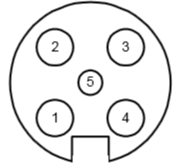This is the general information on the bus used by B&G (Brookes and Gatehouse) H2000 and H3000 systems (and probably also the H1000)

## Fastnet cable

The system is terminated by a 100 ohm resistor at each end, total resistance when measured between White and Green should be 50 ohms

The cable is using 4 strands and the screen on the H2000 and H3000 system.
RED     = 12 Volts DC
BLACK = 0 Volts
WHITE = Network Data +ve
GREEN = Network Data -ve

For H1000, a connector is used, front view of male connector:
1 = 12V
2 = Busy
3 = Fastnet-
4 = Fastnet+
5 = 0V## Fastnet Bus specifications

(Thanks to Tom Lafleur for all the information)
The bus is on a 28k8 speed, with odd parity and 2 stopbits
Green and White are the data lines, together with a 12V and a Ground signal.

Data is sent on a system which looks a bit like CAN.

Complete layout of a Serial TTL to Fastnet schematic (thanks to Tom for all the work) also be aware to change the terminators on the network from 100 ohm to 120-180 ohm. This is to make different kinds of CAN receivers to work. total Resistance should be 60-90 ohm (instead of the normal 50 ohm)

Basic layout of a data frame is this:TO & FROM: list of source and destination node addresses
SIZE: size of data, without headers and checksums
COMMAND: list of all commands, mostly 0x01 is used
HEADER CHECKSUM: this is 0x100 - the total of all the bytes in het header modulo 8 (or: header data modulo 8 + checksum  = 0)
CHANNELNR: list of all the channels being used
FORMAT: this is the format byte, determines decimal dot and format
DATA: mostly 2 or 4 bytes
FULL CHECKSUM: this is 0x100 minus all the bytes modulo 8 (or: all data modulo 8 + checksum = 0)

## Fastnet demo decode

This is an example string recorded on the bus:
ff 05 14 01 e7    1d 61 00 58    1c 51 01 df   87 06 00 be e8 e8  86 06 00 be e8 e8 a8

split it into header and checksum parts:
ff 05 14 01 e7    1d 61 00 58    1c 51 01 df   87 06 00 be e8 e8  86 06 00 be e8 e8 a8
05 = from wind/depth
14 = data in body part, 14hex = 20 dec = 20 databytes
01 = fastnet command, general data send
e7 = checksum header (header data modulo 8 + checksum  = 0)
a8 = full frame checksum(all data modulo 8 + checksum = 0)

decode body parts
the first byte is the channel, the second is a mask to display the data? the rest is data
ff 05 14 01 e7            header
1d 61 00 58              1d = air temp C, format = 61, data = 0058 hex  ==  88dec  ==  88/10 =  8.8 degrees celsius
1c 51 01 df               1c = air temp F, format = 51, data =  01df hex  == 479dec  == 479/10 = 47.9 degrees fahrenheit (= 8.8 degrees celsius)
87 06 00 be e8 e8      87 = barometric pressure, format = 06, raw data is 4 letters, value " Off"
86 06 00 be e8 e8      86 = barometric pressure trend, format = 06, raw data is 4 letters, value " Off"
a8                               checksum
more examples
41 91 02 71           41 = Boatspeed, format = 91, data = 0271 hex = 625 decimal =  625/100 = 6.25 knots
4d 61 00 04           4d = AWS knots, format = 61,  0004 hex = 4 dec  = 04 knots
4f 61 00 02           4f  = AWS m/s,   format = 61,  0002 hex = 2 dec  =  02 m/s (= 4 knots)

format byte
the format byte (e.g. 61) is a bit pattern, so transform this hex value to binary 61HEX = 01 10 00 01
divide the binary value in this format ZZ YY XXXX
ZZ = 01
YY = 10
XXXX = 0001

The ZZ value is the divider (or the place of the decimal point)
00 = xxxx
01 = xxx.x = divide by 10
10 = xx.xx = divide by 100
00 = x.xxx = divide by 1000

the YY value is the number of leading zero's (or the total number of digits visible)
00 = xxxx
01 = xxx
10 = xx
11 = x

The XXXX value is the formatter for different types of data (value, time, segment code for displaying text), check out the excel sheet
0000 =
0001 = normal value in the next 2 bytes
0010 =
0011 =
0100 =
0101 = xx:xx = timer
0110 =
0111 =
1000 =
1001 =
1010 =
1011 =
1100 =
1101 =
1110 =
1111 =